# Tag Archives | Minitab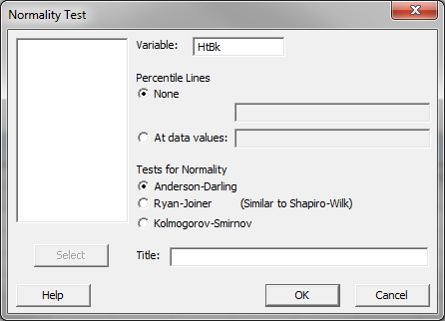## Process Capability with Minitab

What is Process Capability? Process capability measures how well the process performs to meet given specified outcome. It indicates the conformance of a process to meet given requirements or specifications. Capability analysis helps to better understand the performance of the process with respect to meeting customer’s specifications and identify the process improvement opportunities. Process Capability […]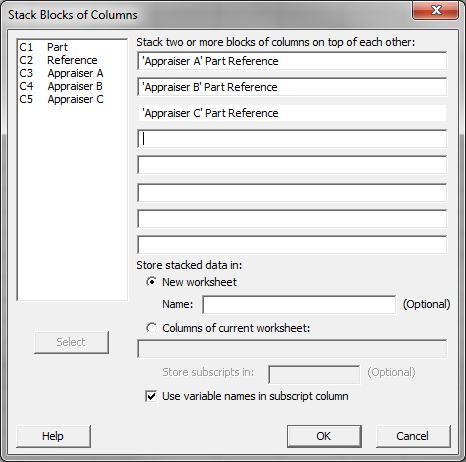## Attribute MSA with Minitab

Use Minitab to Implement an Attribute MSA Data File: “Attribute MSA” tab in “Sample Data.xlsx” (an example in the AIAG MSA Reference Manual, 3rd Edition). Steps in Minitab to run an attribute MSA: Step 1: Reorganize the original data into four new columns (i.e., Appraiser, Assessed Result, Part, and Reference). Click Data → Stack → […]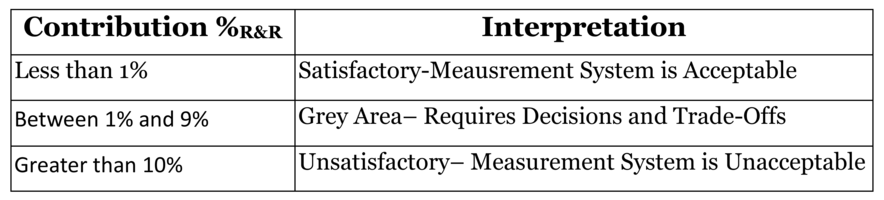## Variable Gage R&R with Minitab

What is Variable Gage R&R? Whenever something is measured repeatedly or by different people or processes, the results of the measurements will vary. Variation comes from two primary sources: We can use a variable Gage R&R to conduct a measurement system analysis to determine what portion of the variability comes from the parts and what […]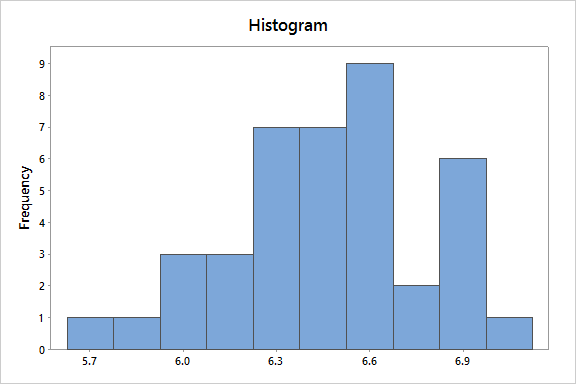## Histogram Rendering with Minitab

What is a Histogram? A histogram is a graphical tool to present the distribution of the data. The X axis represents the possible values of the variable and the Y axis represents the frequency of the value occurring. This graphical summary consists of adjacent rectangles erected over intervals with heights equal to the frequency density of […]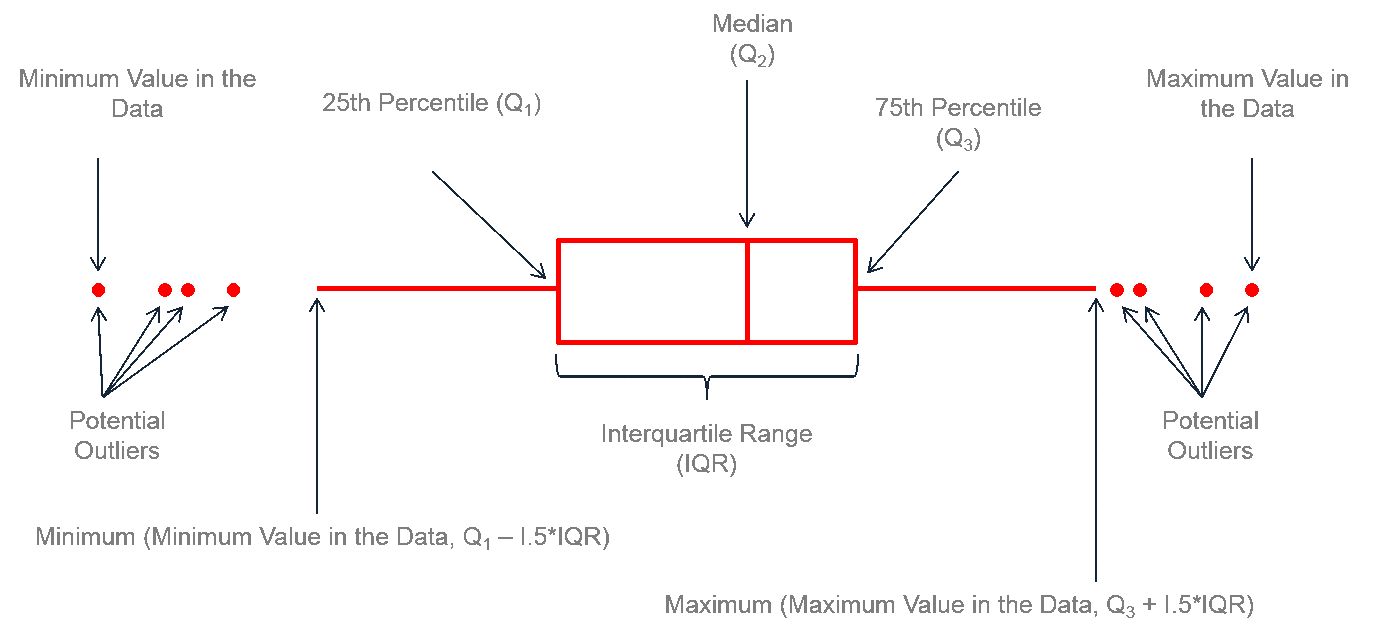## Box Plot with Minitab

What is a Box Plot? A box plot is a graphical method to summarize a data set by visualizing the minimum value, 25th percentile, median, 75th percentile, the maximum value, and potential outliers. A percentile is the value below which a certain percentage of data fall. For example, if 75% of the observations have values […]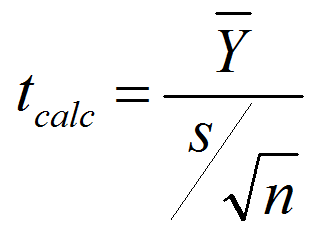## One Sample t Test with Minitab

What is a t Test? In statistics, a t test is a hypothesis test in which the test statistic follows a Student’s t distribution if the null hypothesis is true. We apply a one sample t test when the population variance (σ) is unknown and we use the sample standard deviation (s) instead. A hypothesis […]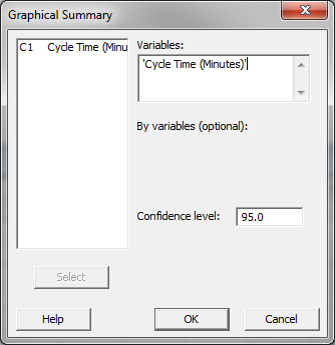## Central Limit Theorem with Minitab

What is Central Limit Theorem? The Central Limit Theorem is one of the fundamental theorems of probability theory. It states a condition under which the mean of a large number of independent and identically-distributed random variables, each of which has a finite mean and variance, would be approximately normally distributed. Let us assume Y1, Y2 […]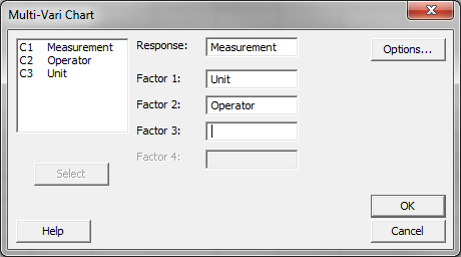## Multi Vari Analysis with Minitab

What is Multi Vari Analysis? Multi Vari analysis is a graphic-driven method to analyze the effects of categorical inputs on a continuous output. It studies how the variation in the output changes across different inputs and helps us quantitatively determine the major source of variability in the output. Multi-Vari charts are used to visualize the […]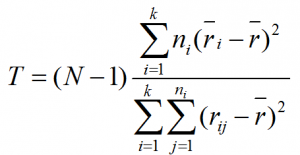## Kruskal Wallis with Minitab

Kruskal Wallis One-Way Analysis of Variance The Kruskal Wallis one-way analysis of variance is a statistical hypothesis test to compare the medians among more than two groups. It is an extension of Mann–Whitney test. While the Mann–Whitney test allows us to compare the samples of two populations, the Kruskal–Wallis test allows us to compare the […]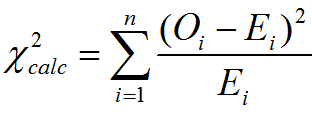## Chi Square Test with Minitab

Chi Square (Contingency Tables) We have looked at hypothesis tests to analyze the proportion of one population vs. a specified value, and the proportions of two populations, but what do we do if we want to analyze more than two populations? A chi-square test is a hypothesis test in which the sampling distribution of the […]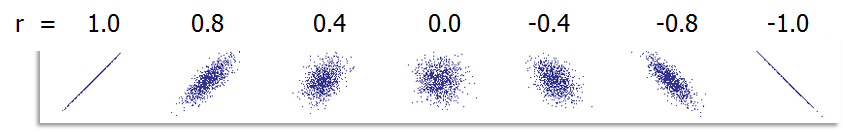## Correlation Coefficient with Minitab

Pearson’s Correlation Coefficient Pearson’s correlation coefficient is also called Pearson’s r or coefficient of correlation and Pearson’s product moment correlation coefficient (r), where r is a statistic measuring the linear relationship between two variables. What is Correlation? Correlation is a statistical technique that describes whether and how strongly two or more variables are related. Correlation [...]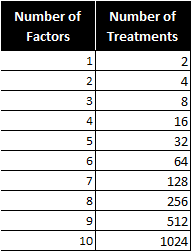## Fractional Factorial Designs with Minitab

What Are Fractional Factorial Experiments? In simple terms, a fractional factorial experiment is a subset of a full factorial experiment. Fractional factorials use fewer treatment combinations and runs. Fractional factorials are less able to determine effects because of fewer degrees of freedom available to evaluate higher order interactions. Fractional factorials can be used to screen [...]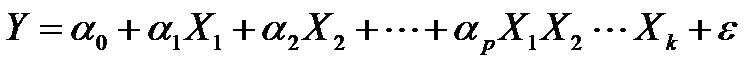## Full Factorial DOE with Minitab

What is a Full Factorial DOE? In a full factorial experiment, all of the possible combinations of factors and levels are created and tested. For example, for two-level design (i.e.each factor has two levels) with k factors, there are 2k possible scenarios or treatments. Two factors, each with two levels, we have 22 = 4 treatments [...]## Logistic Regression with Minitab

What is Logistic Regression? Logistic regression is a statistical method to predict the probability of an event occurring by fitting the data to a logistic curve using logistic function. The regression analysis used for predicting the outcome of a categorical dependent variable, based on one or more predictor variables. The logistic function used to model [...]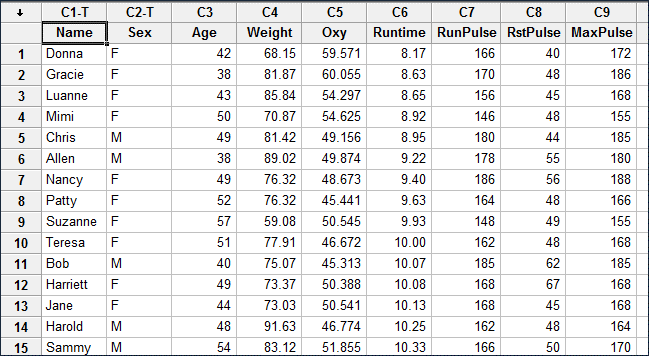## Stepwise Regression with Minitab

What is Stepwise Regression? Stepwise regression is a statistical method to automatically select regression models with the best sets of predictive variables from a large set of potential variables. There are different statistical methods used in stepwise regression to evaluate the potential variables in the model: F-test T-test R-square AIC Three Approaches to Stepwise Regression [...]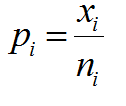## P Chart with Minitab

What is a P Chart? The P chart plots the percentage of defectives in one subgroup as a data point. It considers the situation when the subgroup size of inspected units is not constant. The underlying distribution of the P-chart is binomial distribution. P Chart Equations Data Point:         Center Line:   [...]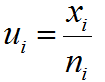## U Chart with Minitab

What is a U Chart? The U chart is a type of control chart used to monitor discrete (count) data where the sample size is greater than one, typically the average number of defects per unit. Defect vs. Defective Remember the difference between defect and defective? A defect of a unit is the unit’s characteristic [...]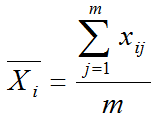## XBar R Chart with Minitab

Xbar R Chart The Xbar R chart is a control chart for continuous data with a constant subgroup size between two and ten. The Xbar chart plots the average of a subgroup as a data point. The R chart plots the difference between the highest and lowest values within a subgroup as a data point. [...]